### Fractions-Solutions Ex-7.2

CBSE Class –VI Mathematics
NCERT Solutions
Chapter 7 Fraction (Ex. 7.2)

Question 1. Draw number lines and locate the points on them:
(a)$\frac{1}{2},\frac{1}{4},\frac{3}{4},\frac{4}{4}$
(b)$\frac{1}{8},\frac{2}{8},\frac{3}{8},\frac{7}{8}$
(c)$\frac{2}{5},\frac{3}{5},\frac{8}{5},\frac{4}{5}$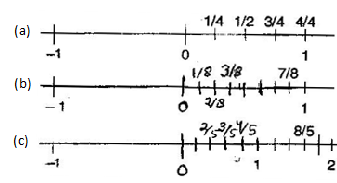Question 2. Express the following fractions as mixed fractions:
(a)$\frac{20}{3}$   (b)$\frac{11}{5}$  (c)$\frac{17}{7}$  (d)$\frac{28}{5}$   (e)$\frac{19}{6}$   (f)$\frac{35}{9}$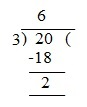$\frac{20}{3}=6\frac{2}{3}$
(b)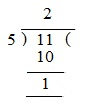$\therefore$$\frac{11}{5}=2\frac{1}{5}$
(c)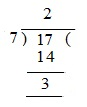$\therefore \frac{17}{7}=2\frac{3}{7}$
(d)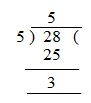$\therefore$$\frac{28}{5}=5\frac{3}{5}$
(e)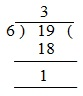$\frac{19}{6}=3\frac{1}{6}$
(f)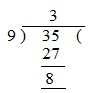$\therefore$$\frac{35}{9}=3\frac{8}{9}$
Question 3. Express the following as improper fractions:
(a)$7\frac{3}{4}$  (b)$5\frac{6}{7}$  (c)$2\frac{5}{6}$  (d)$10\frac{3}{5}$  (e)$9\frac{3}{7}$  (f)$8\frac{4}{9}$
Answer: (a) $7\frac{3}{4}=\frac{\left(7×4\right)+3}{4}=\frac{28+3}{4}=\frac{31}{4}$
(b) $5\frac{6}{7}=\frac{\left(5×7\right)+6}{7}=\frac{35+6}{7}=\frac{41}{7}$
(c) $2\frac{5}{6}=\frac{\left(2×6\right)+5}{6}=\frac{12+5}{6}=\frac{17}{6}$
(d) $10\frac{3}{5}=\frac{\left(10×5\right)+3}{5}=\frac{50+3}{5}=\frac{53}{5}$
(e) $9\frac{3}{7}=\frac{\left(9×7\right)+3}{7}=\frac{63+3}{7}=\frac{66}{7}$
(f)